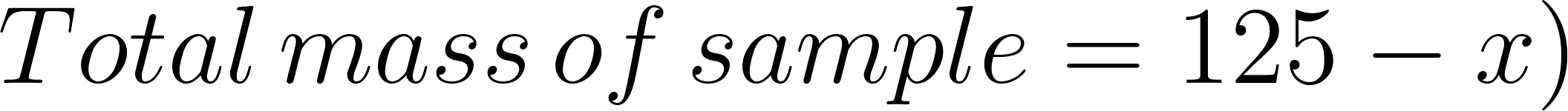×
Get Full Access to Introductory Chemistry - 5 Edition - Chapter 13 - Problem 129p
Get Full Access to Introductory Chemistry - 5 Edition - Chapter 13 - Problem 129p

×

# Solved: A 125-g sample contains only glucose (C6H12O6) and sucrose (C12H22O11). When theISBN: 9780321910295 34

## Solution for problem 129P Chapter 13

Introductory Chemistry | 5th Edition

• Textbook Solutions
• 2901 Step-by-step solutions solved by professors and subject experts
• Get 24/7 help from StudySoup virtual teaching assistantsIntroductory Chemistry | 5th Edition

4 5 1 393 Reviews
15
5
Problem 129P

A 125-g sample contains only glucose $$\mathrm{(C_6H_{12}O_6)}$$ and sucrose $$\mathrm{(C_{12}H_{22}O_{11})}$$. When the sample is added to 0.500 kg of pure water, the resulting solution has a freezing point of $$\mathrm{-1.75~^\circ C}$$. What were the masses of glucose and sucrose in the original sample?

Equation Transcription:Text Transcription:

(C_6H_{12}O_6)

(C_{12}H_{22}O_{11})

-1.75 ^oC

Step-by-Step Solution:

Step 1 of 4

From the given,

Mass of  sample = 125g

Let the mass of glucose = x

Molar mass of glucose = 180 g/mol

Molar mass of sucrose = 342.34 g/molStep 2 of 4

Step 3 of 4

## Discover and learn what students are asking

Statistics: Informed Decisions Using Data : The Binomial Probability Distribution
?Explain how the value of p, the probability of success, affects the shape of the distribution of a binomial random variable.

Statistics: Informed Decisions Using Data : The Normal Approximation to the Binomial Probability Distribution
?Suppose X is a binomial random variable. To approximate P(X < 5), compute _________ .

Statistics: Informed Decisions Using Data : Inference about the Difference between Two Medians: Dependent Samples
?In Problems 3–10, use the Wilcoxon matched-pairs signedranks test to test the given hypotheses at the a = 0.05 level of significance. The dependent sa

Unlock Textbook Solution